#### 期刊菜单

Research on Evacuation Path Planning Based on Indoor Hypergraph Network Model
DOI: 10.12677/GST.2020.83015, PDF, HTML, XML, 下载: 198  浏览: 433  国家科技经费支持

Abstract: In order to study the organization and visualization of indoor network models for emergency evacuation scenarios, this paper introduces hypergraph theory on the basis of graph theory, and designs the data organization method of Hypergraph Network Model, and describes semantic in-formation of the path complexity, path congestion degree, and emergency events involved in emergency evacuation scenarios. Indoor Hypergraph Network Model is constructed and applied to indoor emergency path planning research. The feasibility of the Indoor Hypergraph Network Model was verified through experiments, and based on this model, the indoor emergency evacuation path planning algorithm group experiment was carried out. Experimental results show that the indoor emergency evacuation path planning algorithm in this paper can effectively synthesize the effects of path complexity, path congestion degree, and emergencies, and obtain comprehensive and reasonable path planning results.

1. 引言

2. 室内超网络模型

2.1. 超图理论

${e}_{i}\ne \varphi \left(i=1,2,3,\cdots ,m\right)$${\cup }_{i=1}^{m}{e}_{i}=V$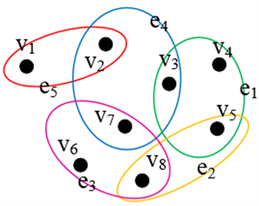Figure 1. Example of undirected hypergraph

2.2. 室内超网络结构

1) 超网络层(Hyper-floor)：即各楼层，将整个建筑物划分为不同的楼层结构，由水平结构和垂直结构组成。

2) 区块网络层(Hyper-block)：即楼层中的区块域，是对同一楼层中的区域划分，划分依据一般是区域功能或者位置排列特点。

3) 空间对象(Objects)，具体包括：

① 封闭空间(Room)：由墙体、门窗、天花板、地面等组成的封闭或半封闭空间。

② 通道(Corridor)：具有连通其他室内独立空间对象的功能。

③ 门(Door)：控制封闭空间和通道之间的连通性。

④ 楼层通道(F-connection)：连通不同楼层的室内结构，如电梯、楼梯、扶梯等。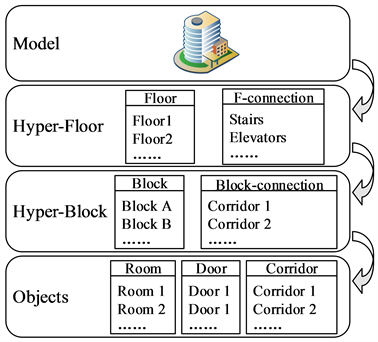Figure 2. Schematic diagram of indoor super network structure division

2.3. 室内语义表达

1) 路径复杂度

$\text{Rout_complexity}=\left\{\text{HyperEdge_degrees}\left(\text{Node_degree}\right)\right\}$ (1)

2) 路径拥挤度

$\text{Rout_crowd}=\left\{\text{cr_type},\text{cr_range}\right\}$ (2)

3) 突发情况

$\text{Rout_accident}=\left\{\text{ac_range}\right\}$ (3)

4) 路径复杂程度函数

a) 路径复杂度函数

${F}_{cm}\left({V}_{m}\right)=\text{Hyper_degrees}\left(\text{Node_degree}\right)$ (4)

b) 路径拥挤度函数

${F}_{cw}\left({v}_{i},{v}_{j}\right)=\beta D\left({v}_{i},{v}_{j}\right)$ (5)

c) 突发事件函数

${F}_{ac}\left({V}_{n}\right)=\left\{\begin{array}{l}1,\text{\hspace{0.17em}}\text{\hspace{0.17em}}\text{\hspace{0.17em}}\text{\hspace{0.17em}}\text{\hspace{0.17em}}\text{\hspace{0.17em}}\text{\hspace{0.17em}}{V}_{n}中节点可通行\\ \to 0,\text{\hspace{0.17em}}\text{\hspace{0.17em}}{V}_{n}中节点不可通行\end{array}$ (6)

d) 超边权值函数

${D}^{\prime }=\frac{{\alpha }_{cm}{F}_{cm}\left({v}_{i},{v}_{j}\right)+{\alpha }_{cw}{F}_{cw}\left({V}_{m}\right)}{{\alpha }_{ac}{F}_{ac}\left({V}_{n}\right)},\text{\hspace{0.17em}}\sum {\alpha }_{k}=1,\text{\hspace{0.17em}}{\alpha }_{k}\in \left(0,1\right),\text{\hspace{0.17em}}k\in \left\{\text{CM},\text{CW},\text{AC}\right\}$ (7)

${D}^{*}=\frac{{\alpha }_{cm}{F}_{cm}^{\ast }\left({v}_{i},{v}_{j}\right)+{\alpha }_{cw}{F}_{cw}^{\ast }\left({V}_{m}\right)}{{\alpha }_{ac}{F}_{ac}^{\ast }\left({V}_{n}\right)}$ (8)

3. 应急疏散路径规划方法

1) 在室内超网络模型的基础上，构建完整的室内网络数据。

2) 确定起始点S和目标点E，定义一个集合(CloseList)用于存放已遍历点；再定义一个集合(RoutList)用于存放最优路径，并初始化集合。

3) 根据超网络模型中节点和超边的集合和属性信息，计算改进的超边权值D*

4) 判断S和E是否同层，若是则采用同层算法；反之则采用分层算法。

5) 将计算得到的RoutList集合结果输出并可视化显示。至此，算法结束。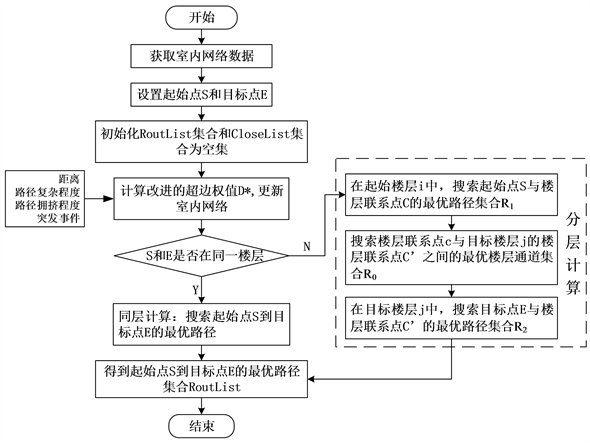Figure 3. Flow chart of the improved Dijkstra algorithm

4. 室内超网络模型验证及分析

4.1. 实验区域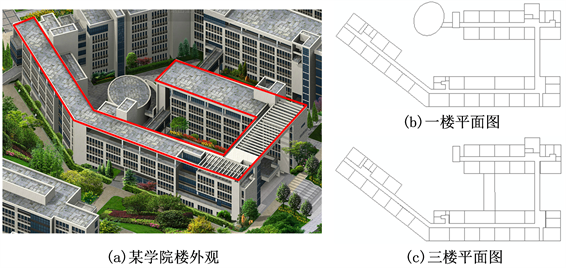Figure 4. Study area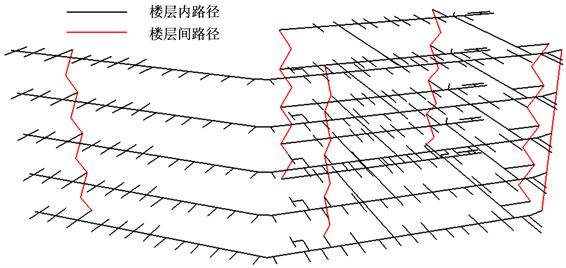Figure 5. Hypergraph ModelTable 1. Comparison of Hyepergraph Model and classic Node-Arc Model

4.2. 疏散模拟Table 2. Hierarchical division of path congestion and coefficient values

4.3. 面向应急疏散的路径规划Table 3. Emergency evacuation planning experiment

1) 实验一

2) 实验二

3) 实验三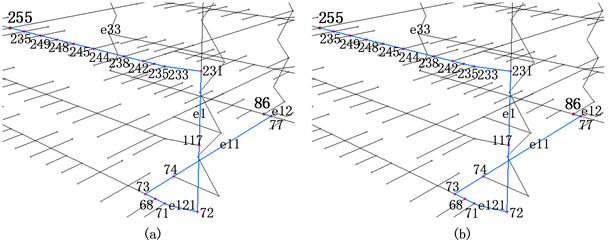Figure 6. Results of Experiment 1 (a) and Experiment 2 (b)

4) 实验四Figure 7. Results of Experiment 3Figure 8. Results of Experiment 4 (a) and Experiment 5 (b)

5) 实验五

5. 结语

“十三五”国家重点研发计划(室内混合智能定位与室内GIS技术) (2016YFB0502102)。

  Tashakkori, H., Rajabifard, A., Kalantari, M., et al. (2016) Indoor Incident Situation Awareness Using a 3D In-door/Outdoor Spatial City Model. International Conference on Information and Communication Technologies for Disaster Management, Rennes, 30 November-2 December 2015, 240-245. https://doi.org/10.1109/ICT-DM.2015.7402050  Lee, H., Hong, S.G., Lee, H. and Lee, K.B. (2019) An In-formation Model and System for Fire Management Based on Integration of Fire Safety and Spatial Information. The Journal of Korean Institute of Communications and Information Sciences, 44, 1892-1904. https://doi.org/10.7840/kics.2019.44.10.1892  Kwan, M.-P. and Lee, J. (2003) Emergency Response after 9/11: The Potential of Real-Time 3D GIS for Quick Emergency Response in Micro-Spatial Environments. Computers, Environment and Urban Systems, 29, 93-113. https://doi.org/10.1016/j.compenvurbsys.2003.08.002  Li, X., Zhang, X.H. and Tan, L. (2007) Assisting Video Surveillance in Micro-Spatial Environments with a GIS Approach. Geospatial Information Technology and Applications, 6754.  Mandloi, D. and Thill, J.C. (2010) Object-Oriented Data Modeling of an Indoor/Outdoor Urban Transportation Network and Route Planning Analysis. In: Jiang, B. and Yao, X., Eds., Geospatial Analysis and Model-ling of Urban Structure and Dynamics, Springer, Netherlands, 197-220. https://doi.org/10.1007/978-90-481-8572-6_11  Zhou, Y., Zeng, G., Huang, Y., et al. (2017) Indoor Space Location Model Based on Location Service. International Archives of the Photogrammetry Remote Sensing & Spatial Information Sciences, XLII-4/W7, 49-53. https://doi.org/10.5194/isprs-archives-XLII-4-W7-49-2017  程全胜. 超图路径求解算法及其应用[D]: [硕士学位论文]. 武汉: 华中科技大学, 2008.  张大坤, 任淑霞. 超图可视化方法研究综述[J]. 计算机科学与探索, 2018, 12(11): 1701-1717.  周艳, 陈红, 张叶廷, 黄悦莹, 张鹏程, 杨卫军. 动态环境感知的多目标室内路径规划方法[J]. 西南交通大学学报, 2019, 54(3): 611-618, 632.  王行风. 面向室内外一体化寻径的道路网络空间感知层次建模方法[J]. 测绘科学技术, 2018, 6(2): 141-150.  Lee, J. (2014) Route Optimization for Emergency Evacuation and Response in Disaster Area. Journal of the Korean Society of Civil Engineers, 34, 617-626. https://doi.org/10.12652/Ksce.2014.34.2.0617  丁雨淋, 何小波, 朱庆, 林珲, 等. 实时威胁态势感知的室内火灾疏散路径动态优化方法[J]. 测绘学报, 2016, 45(12): 1464-1475.  李瑞琪. 三维蚁群算法的实现与疏散路径优化研究[D]: [硕士学位论文]. 沈阳: 沈阳航空航天大学, 2012.  赵俊波. 基于改进粒子群优化算法的人员疏散问题研究[D]. 鞍山: 辽宁科技大学, 2016.